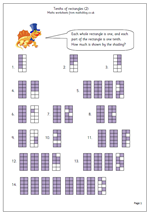# Year 4 decimalsOne of the best ways to introduce decimals is by working with money which shows the idea of tenths (10p) and hundredths (1p). Another good way is to use shading of shapes and this is the second page which does this. A series of rectangles is shown, each divided into ten equal parts: each part being one tenth. This is a very clear illustration of what tenths are and children should find it relatively easy to write down how much is shown: this could also be used on a whiteboard as a whole class activity at the start of the lesson.

It could also be used to show that 1/10 is the same as 0.1, 2/10 the same as 0.2 etc.

This is the second page of Tenths of Rectangles and can be found in our year 4 Counting and Numbers category.

Tenths of rectangles (2)